Courses

# Test: Mensuration

## 20 Questions MCQ Test Topic-wise Past Year Questions for CAT | Test: Mensuration

Description
This mock test of Test: Mensuration for CAT helps you for every CAT entrance exam. This contains 20 Multiple Choice Questions for CAT Test: Mensuration (mcq) to study with solutions a complete question bank. The solved questions answers in this Test: Mensuration quiz give you a good mix of easy questions and tough questions. CAT students definitely take this Test: Mensuration exercise for a better result in the exam. You can find other Test: Mensuration extra questions, long questions & short questions for CAT on EduRev as well by searching above.
QUESTION: 1

### If the rectangular faces of a brick have their diagonals in the ratio 3 : 2√3 : √15 , then the ratio of the length of the shortest edge of the brick to that of its longest edge is (2019)

Solution:

Let the edges of the brick be a, b, and c such that
a < b < c
a2 + b2 = 32 = 9 ...(i)
a2 + c2 = ( 2√3 )2 = 12 ...(ii)
b2 + c2 = ( √15 )2 = 15 ...(iii)
Adding all three equations. We get
2(a2 + b2 + c2) = 9 + 12 + 15 = 36
a2 + b2 + c2 = 18 ...(iv)
From (i) and (iv), c2 = 18 – 9 ⇒ c = 3
From (iii) and (iv) a2 = 18 – 15 ⇒ a = 3
Therefore, required ratio =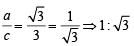QUESTION: 2

### Given an equilateral triangle T1 with side 24 cm, a second triangle T2 is formed by joining the midpoints of the sides of T1. Then a third triangle T3 is formed by joining the midpoints of the sides of T2. If this process of forming triangles is continued, the sum of the areas, in sq cm, of infinitely many such triangles T1, T2, T3,... will be (2018)

Solution:

An equilateral triangle formed by joining the midpoints of the sides of a given equilateral triangle will have its side equal to half the side of the given equilateral triangle.
Now, side of T1 = 24 cm
Side of T2 = 12 cm
Side of T3 = 6 cm
and so on.
Sum of the areas of all the triangles =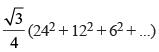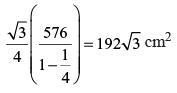QUESTION: 3

### Let ABCD be a rectangle inscribed in a circle of radius 13 cm. Which one of the following pairs can represent, in cm, the possible length and breadth of ABCD? (2018)

Solution:

Let the length and the breadth of the rectangle be l and b respectively.
Diameter of the circle = Diagonal of the rectangle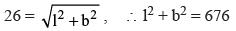Hence, possible values of l and b are 24 and 10 respectively.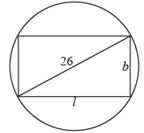QUESTION: 4

A right circular cone, of height 12 ft, stands on its base which has diameter 8 ft. The tip of the cone is cut off with a plane which is parallel to the base and 9 ft from the base. With π = 22/7, the volume, in cubic ft, of the remaining part of the cone is

(2018)

Solution:

The radius of the cone is 4 feet.
The tip of the cone is a cone of height 3 feet.
By similarity, its radius is 1 foot.
The volume of the remaining part of the cone
= Volume of the cone – Volume of the tip of the cone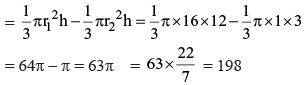QUESTION: 5

A solid metallic cube is melted to form five solid cubes whose volumes are in the ratio 1 : 1 : 8 : 27 : 27. The percentage by which the sum of the surface areas of these five cubes exceeds the surface area of the original cube is nearest to

(2017)

Solution:

Volume ratio of 5 smaller and original cube = 1:1 : 8 : 27 : 27 : 64
Side ratio of 5 smaller and original cube = 1 : 1 : 2 : 3 : 3 : 4
Surface area ratio of 5 smaller and original cube = 1 : 1 : 4 : 9 : 9 : 16
Sum of smaller cube (surface area) = 24 parts.
Big cube = 16 parts.
The sum is 50% greater.

QUESTION: 6

A regular polygon has an even number of sides. If the product of the length of its side and the distance between two opposite sides is 1/4 th of its area, find number of sides it has

(2016)

Solution:

Let the number of sides be 2n. Let the length of the side of S and the length of the perpendicular from the centre to each side be P. Since the number of sides is even, the opposite sides will be parallel and the distance between any two opposite sides is equal to 2P.
Also, area of the polygon (A) =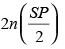...(i)
According to question,
S(2P) = A/4 or SP = A/8
∴ (1) ⇒ A = n (A/8)
⇒ n = 8 or 2n = 16
∴ number of sides = 16.

QUESTION: 7

A field is in the form of a rectangle of dimension 24 m × 56 m. There is 2700 m of fencing that is available. The field has to be divided into many identical smaller square plots, having integral sides (in metres), each of which is to be fenced. Find the side of each of the square plots such that the fencing material that is left out is minimum.

(2015)

Solution:

Here, HCF of 24 and 56 = 8 then, the side of the identical square plots = factors of 8.
The factors of 8 are 1, 2, 4 and 8
If side of the square plot is 1 m. the length of fencing material required is (25 × 56 + 57 × 24) = 2768 m
But 2768 m > 2700 m. (So, It is not possible)
If side of the square plot is 2 m. the length of fencing material required is
(29 × 24+13 × 56) =1464m < 2700 m
∴ minimum fencing material to be left the side  of identical square plot = 2m.

QUESTION: 8

ABCD is an isosceles trapezium with BC = AD = 10 units, AB = 2 units and CD = 14 units. The mid-points of the sides of the trapezium are joined to form a quadrilateral PQRS. Find the ratio of the area of the circle inscribed in the quadrilateral PQRS to the area of trapezium ABCD.

(2015)

Solution: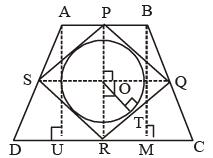PQRS is a rhombus, so the centre of the inscribed circle will be the center of the rhombus PQRS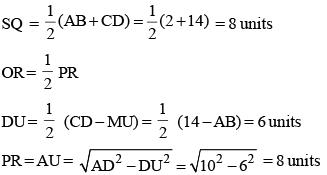PR = SQ = 8 units
⇒ PQRS is a square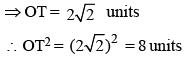Area of trapezium =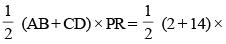8 = 64 sq. unit.
Required ratio =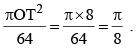QUESTION: 9

The smallest possible circle touching two opposite sides of a rectangle is cut-out from a rectangle of area 60 sq. units. If the area of this circle is 3/2 times the area left out in the rectangle, find the length of the smaller side of the rectangle.

(2015)

Solution: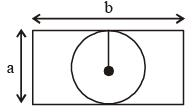Let length of smaller side of rectangle = a
∴ Radius = a/2 of circle
According to question,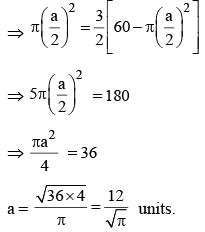So, length of smaller side of rectangle =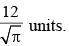QUESTION: 10

The top and bottom radii of a frustrum of a solid cone are 3 cm and 6 cm respectively. Its height is 8 cm. There is a conical cavity of height 3 cm and radius 6 cm at the bottom. The amount of material in the solid is

(2014)

Solution: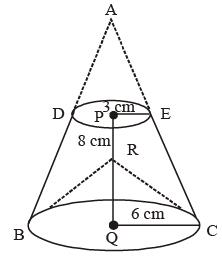Here, ABC represents cone; DEBC represents frustum which was made by cone and BRC represents conical cavity.
So, Volume of material = cone ABC – (cone ADE + conical cavity of BRC).
Now, ΔAPE and ΔAQC are similary,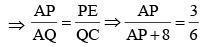⇒ So, AP = 8 cm.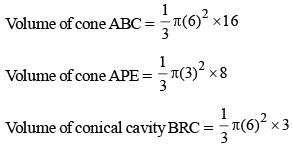Required volume of solid =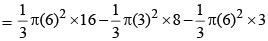= 132π cm3.

QUESTION: 11

The length of the hypotenuse of a right-angled triangle is 240 units. The perimeter of the given triangle is a perfect square. If the perimeter of the given triangle is greater than 550 units, then which of the following can be the length of a side of the given right-angled triangle?

(2014)

Solution:

Let the length of the other two sides of the triangle be 'x' and 'y' respectively. then,
x2 + y2 = (240)2 (hypotenuse of triangle = 240)
Also, x + y + 240 must be perfect square (because perimeter of triangle is perfect square).
When the two sides other than hypotenuse are equal length than perimeter of right angled triangle is maximized.
Therefore,
The maximum perimeter of right angled triangle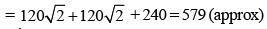Also,
The perimeter of triangle should be greater than twice the length of hypotenuse of triangle.
Then, the perimeter of triangle should be greater than 480 and less than 579. according to question.
Perimeter of triangle should be 484, 529 and 576
(because perimeter is perfect square)
Again, according to question, Perimeter of triangle is greater than 550, So perimeter = 576.
Here, perimeter of triangle = 576.
According to pythogorean triples.
The value of x and y will be 192 and 144 units.

QUESTION: 12

How many rectangles with integral sides are possible where the area of the rectangle equals the perimeter of the rectangle?

(2014)

Solution:

Let the length and breadths of rectangle be a units and b units.
According to question,
ab = 2a + 2b
a = 2b / (b – 2)
Here, Two cases are possible
(i) a = 4, b = 4
(ii) a = 3 and b = 6
Thus, two different rectangles are possible.

QUESTION: 13

A spherical ball of the maximum possible volume is placed inside a right-circular cone of height ‘h’ units. If the radius of the base of the cone is equal to h/ √3 units, then the ratio of the volume of the sphere to that of the cone is

(2013)

Solution: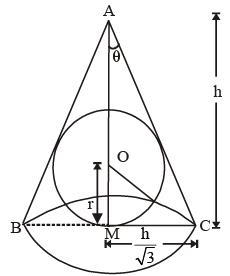Let the semi vertical angle of the cone be θ.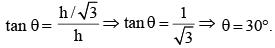The vertical angle of the cone = 60°
Therefore, the triangle ABC is an equilateral triangle.
Therefore, the center (O) of the circle will coincide with the centroid of the triangle ABC.
In triangle ACM, OC is angle bisector of ∠ACM.
∴ ∠OCM = 30°
In ΔOCM,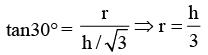Hence, the ratio of the volume of the sphere to that of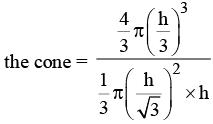∴ Required ratio = 4 : 9

QUESTION: 14

How many triangles can be drawn by joining any three vertices of a pentagon?

(2013)

Solution:

Required number of traingles =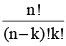Here, n = number of items and
k = number you are picking at time
∴ n = 5, k = 3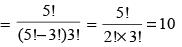QUESTION: 15

A trapezium DEFG is circumscribed about a circle that has centre at C. If DM = 1 cm, GN = 4 cm and the measure of ∠DEF = ∠EFG = 90°, then find the radius of the circle.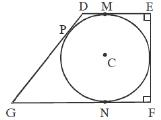(2012)

Solution:

Let, the radius of circle be 'x' cm.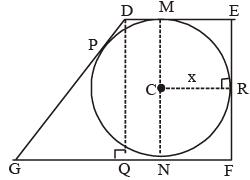∴ CM = CN = CR =  x
Given that GN = 4 cm
∴ GP = 4 cm
Also, DP = DM = QN = 1 cm
∴ GD = 5 cm
GQ = GN – QN = 4 – 1 = 3 cm
In ΔDGQ : DQ =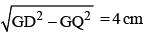⇒ 2x = 4 cm (∵ DQ = MN)
∴  x = 2 cm
∴ radius or circle = 2 cm

QUESTION: 16

A rectangle with perimeter 88 m is partitioned into 5 congruent rectangles, as shown in the diagram given below. The perimeter of each of the congruent rectangles is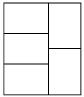(2012)

Solution:

Let the lengths and breaths of each,
Small rectangles be 'x' meter and 'y' meter respectively.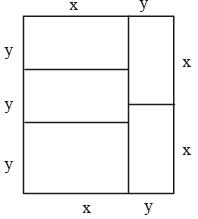∵ Perimeter = 88 m
∴ 5y + 4x = 88 and 3y = 2x
​∴ 5y + 3y × 2 = 88
5y + 6y = 88  ⇒ 11y = 88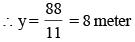​∴ 5 × 8 + 4x = 88
4x = 88 – 40 = 48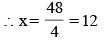∴ Perimeter of each small rectangle = 2 (x + y)
= 2 (12 + 8) = 40 meter.

QUESTION: 17

In the regular hexagon shown below, what is the ratio of the area of the smaller circle to that of the bigger circle?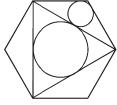(2012)

Solution: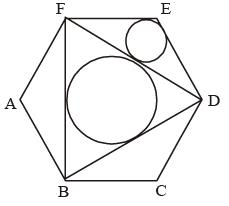Let  EF = s unit
In Δ DEF : DF = 2EF cos30° = √3s
Semi perimeter of D DEF (in units) = S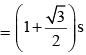Area of Δ DEF (in unit2 = Δ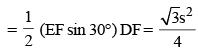Inradius of Δ DEF (in units) = r2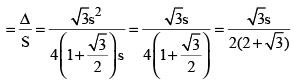In radius of triangle DEF (in units) = r2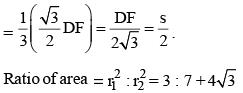QUESTION: 18

A circle of radius 6.5 cm is circumscribed around a right-angled triangle with the sides a, b and c cm where a, b and c are natural numbers. What is the perimeter of the triangle?

(2012)

Solution:

Centre of the circle will be mid-point of the hypotenuse of the triangle.
So, hypotenuse = 2 × 6.5 = 13 cm.
According to Pythagorean triples.
other sides are 5 cm and 12 cm because all sides are natural number.
∴ So perimeter = 5 + 12 + 13 = 30 cm

QUESTION: 19

From a square piece of card-board measuring 2a on each side of a box with no top is to be formed by cutting out from each corner a square with sides b and bending up the flaps. The value of b for which the box has the greatest volume is

(2011)

Solution:

Since cubic shape will be the greatest volume (as volume = side3)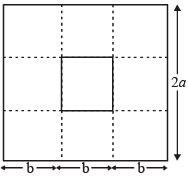Hence, 3b = 2a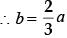QUESTION: 20

A pole has to be erected on the boundary of a circular park of diameter 13 metres in such a way that the difference of its distances from two diametrically opposite fixed gates A and B on the boundary is 7 metres. The distance of the pole from one of the gates is:

(2011)

Solution: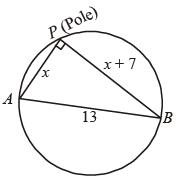In right ΔAPB,
x2 + (x + 7)2 = 132
⇒ x = 5 ∴ x + 7 = 12.